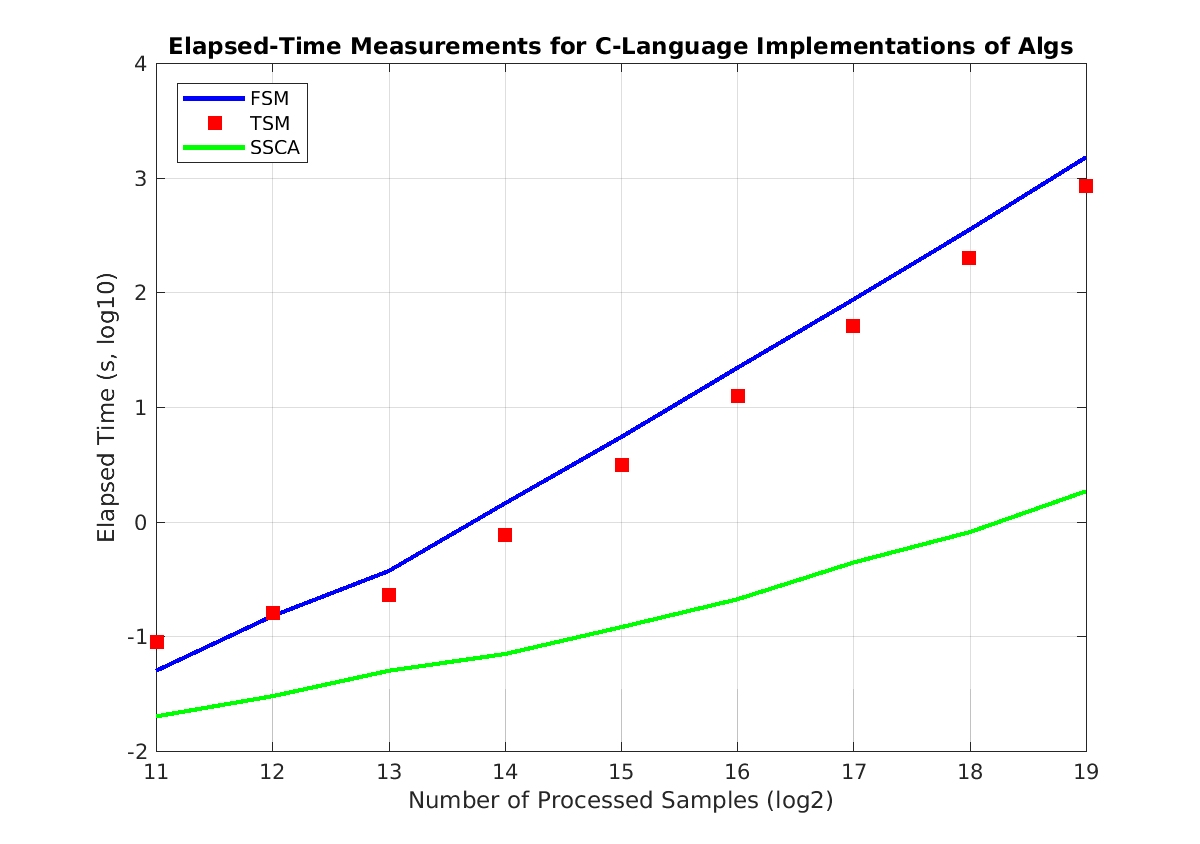# Computational Costs for Spectral Correlation Estimators

Let’s look at the computational costs for spectral-correlation analysis using the three main estimators I’ve previously described on the CSP Blog: the frequency-smoothing method (FSM), the time-smoothing method (TSM), and the strip spectral correlation analyzer (SSCA).

We’ll see that the FSM and TSM are the low-cost options when estimating the spectral correlation function for a few cycle frequencies and that the SSCA is the low-cost option when estimating the spectral correlation function for many cycle frequencies. That is, the TSM and FSM are good options for directed analysis using prior information (values of cycle frequencies) and the SSCA is a good option for exhaustive blind analysis, for which there is no prior information available.

### The Cost of the Frequency-Smoothing Method

Recall that the FSM convolves the cyclic periodogram with a pulse-like smoothing window$g(f)$. The cyclic periodogram is defined by$\displaystyle I^\alpha(t, f) = \frac{1}{N} X(t, f + \alpha/2) X^*(t, f - \alpha/2), \hfill (1)$

for a complex-valued power signal$x(t)$ with sliding$N$-point Fourier transform$X(t, f)$ for the data segment that is centered at time$t$ and having width$N$ samples. A complication occurs when the cycle frequency$\alpha$ is such that$\alpha/2$ is not equal to an integer number of frequency-bin widths$k/N$. This complication is discussed in the FSM post, and isn’t important for our cost analysis.

To obtain an estimate of the SCF, we convolve the cyclic periodogram with the smoothing function$g(f)$:$\hat{S}^\alpha (f) = g(f) \otimes I^\alpha(f) \hfill (2)$

So the cost of the FSM is the sum of the costs for (1) the FFT, (2) computing the cyclic periodogram, and (3) the convolution with$g(f)$. The FFT has approximate cost of$N \log_2(N)$ complex operations, the cyclic periodogram costs approximately$N$ complex operations, and the convolution cost depends on the number of frequency points contained within the width of$g(f)$. In the special case of a rectangular$g(f)$, however, the cost can be reduced to approximately$2N$ operations.

For example, if$N = 32,768$ samples, then the FFT cost is$491,520$, the cyclic periodogram cost is$32,768$, and the smoothing is$65,536$, indicating that the dominant cost is the FFT.$\displaystyle C_{FSM} \approx N \log_2(N) + 3N. \hfill (3)$

### The Cost of the Time-Smoothing Method

In the TSM, multiple relatively short-duration data blocks are used to estimate multiple cyclic periodograms, which are then phase-shifted and averaged. As in the FSM analysis, let the total number of samples to be processed equal$N$. The sub-block length is$M$, so that$K = N/M$ individual cyclic periodograms are averaged.

The cost calculations are straightforward. Each$M$-point FFT costs$M\log_2(M)$ operations, each scaling by the complex-valued scale factor costs$M$ operations, and the summing of the scaled cyclic periodograms costs$(K-1)M \approx N$ operations. So the cost is about$\displaystyle C_{TSM} = K (M \log_2(M)) + (K-1)M + (K-1)M \approx N \log_2(M) + 2N \hfill (4)$

### The Cost of the Strip Spectral Correlation Analyzer

The SSCA employs three major computational steps. The first is a FFT-based channelization step. The size of the FFT is$N_{chan}$ (the number of strips), which is applied to successive$N_{chan}$-long data blocks obtained by sliding forward in time by$L \ge 1$ samples. As before, the total number of processed data samples is$N$. Typically I used$L = 1$ because I do not need to limit the memory requirement for the channelizer output. In this case, there are$N - N_{chan}$ data blocks of size$N_{chan}$ to process through the channelizer, and since$N \gg N_{chan}$ in all cases of practical interest, we’ll approximate this by$N$. So the cost for the channelizer stage is$N$ FFTs of length$N_{chan}$, or$N N_{chan} \log_2 (N_{chan})$.

The second SSCA computation is the multiplication of the original complex-valued data by each of the channelizer outputs, creating$N_{chan}$ new complex-valued sequences of length$N$. This requires$NN_{chan}$ operations.

The final step in the estimation of the spectral correlation function using the SSCA is to apply an$N$-point FFT to each of the$N_{chan}$ sequences obtained from the second step. So this cost is approximately$N_{chan} N \log_2(N).$$\displaystyle C_{SSCA} \approx N N_{chan} \log_2(N_{chan}) + NN_{chan} + N_{chan} N \log_2(N) \hfill (5)$

or$\displaystyle C_{SSCA} \approx NN_{chan} (\log_2(N_{chan}) + \log_2(N) + 1). \hfill (6)$

### The Cost of Computing the Spectral Coherence

Recall that the spectral coherence is the correlation coefficient corresponding to the correlation that is the spectral correlation function:$\displaystyle C_x^\alpha(f) = \frac{S_x^\alpha(f)}{\left[ S_x^0(f+\alpha/2) S_x^0(f-\alpha/2)\right]^{1/2}} \hfill (7)$

For each spectral correlation point estimate$S_x^\alpha(f)$ we have to multiply two PSD values together, take the square root of that product, and then divide the point estimate by the result. Each of those normalization steps costs two multiplications and one square root. We’ll count this as three operations.

### Comparisons

First let’s compare the costs for the three algorithms when we use them to perform exhaustive spectral correlation and spectral coherence analysis. Including the coherence is important because it is the function that is used to do the actual cycle-frequency detection.  Here we take the cost for the FSM and multiply by the number of cycle frequencies that make up the principal domain of the discrete-time/discrete-frequency spectral correlation in the$f$$\alpha$ plane, which we’ll take to be$N.$To verify these trends, I also ran my C-language versions of the three algorithms and measured the elapsed times:Now, what about the case where we just want the spectral correlation function or spectral coherence function (or both) for a single cycle frequency? This might happen, for example,  in the context of the cycle detectors. For the TSM and FSM, we just have the single-cycle-frequency costs described above. But for the SSCA, we realize that we can’t get at the function$S_x^\alpha(f)$ as a function of$f$ without, in general, combining elements multiple strips. We could create another algorithm that doesn’t perform the final$N$-sample FFTs, but instead computes only the particular DFT coefficients that correspond to$S_x^\alpha(f)$ for each$f$, or we can just apply the usual SSCA and extract the needed spectral correlation and coherence values. If we do the latter, we have the cost comparison:I’ll be referring to the content of this post in my upcoming post on tunneling (also see here). So let me know if you see errors or issues!